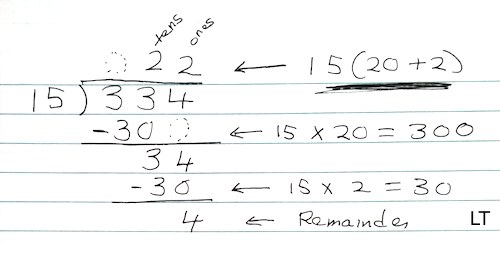## Saturday, July 9, 2016

### Long Division

Standard Algorithm for Division: Grade 3
1. Teach the steps and convergent strategies for the standard algorithm. I assume that you know the steps. Do not teach the partial quotient method.
2. Make sure students have automated the steps. Drill for skill. I assume students have auto recall of multiplication facts.
3. Lastly, explain the reasons why the algorithm works. The illustration below will help you help students understand it better. Repeated subtraction and the distributive property are fundamental ideas in the algorithm.  Students do not need to write an explanation of why the algorithm works.Standard Algorithm for Division. My comments..

The method is repeated subtraction. First 15(20), then (15)(2), or 15(20 + 2). "The distributive axiom helps shorten the number of steps. Without it you would have to subtract 15 from 334 twenty-two times," explains Mary P. Dolciani .

Do not use other methods to verify an answer. Use the regular check.

15(20 + 2) + 4 = the Dividend
300 + 30 + 4 = 334
334 = 334 True
(OR: 15 x 22 + 4)

In the 70s and 80s, many public, parochial, and private schools had taught the standard division algorithm in the second semester of 3rd grade. Multiplication and word problems dominated the 1st semester. Division and word problems dominated the 2nd semester. For example, the 1997 California 3rd-grade math standards included long-division. Some schools still do!

The old California standards, which were adopted in December of 1997, got it right. By the end of 3rd grade, students should know well (be able to apply and perform efficiently) the four standard algorithms for whole numbers (addition, subtraction, multiplication, and long division).

Notes
 Dolciani & Wooton, Book 1, Modern Algebra Structure And Method, 1973 Revised Edition. This is one of the best Algerba-1 textbooks ever.  It also covers trig and vectors, which were used to solve problems.

Note. The properties of numbers give math structure.